# Elly Cooper and the City of Antiquity WalkthroughElly Cooper and the City of Antiquity Walkthrough
* OS: Windows 7 / Vista / XP
* CPU: Pentium 3 1.0 GHz or HigherElly Cooper and the City of Antiquity Walkthrough
* OS: Windows 7 / Vista / XP
* CPU: Pentium 3 1.0 GHz or Higher

## Elly Cooper and the City of Antiquity Walkthrough Introduction:

Elly Cooper and the City of Antiquity is a great hidden object game published by Big Fish Games. This detailed walkthrough includes 160 screenshots and 640 steps, use this walkthrough as a guide when you play the game. Our editors take times to play the game, capture screenshots, mark the interactive area on the image, please share this walkthrough on your facebook or twitter pages and let more hidden object game fans know it.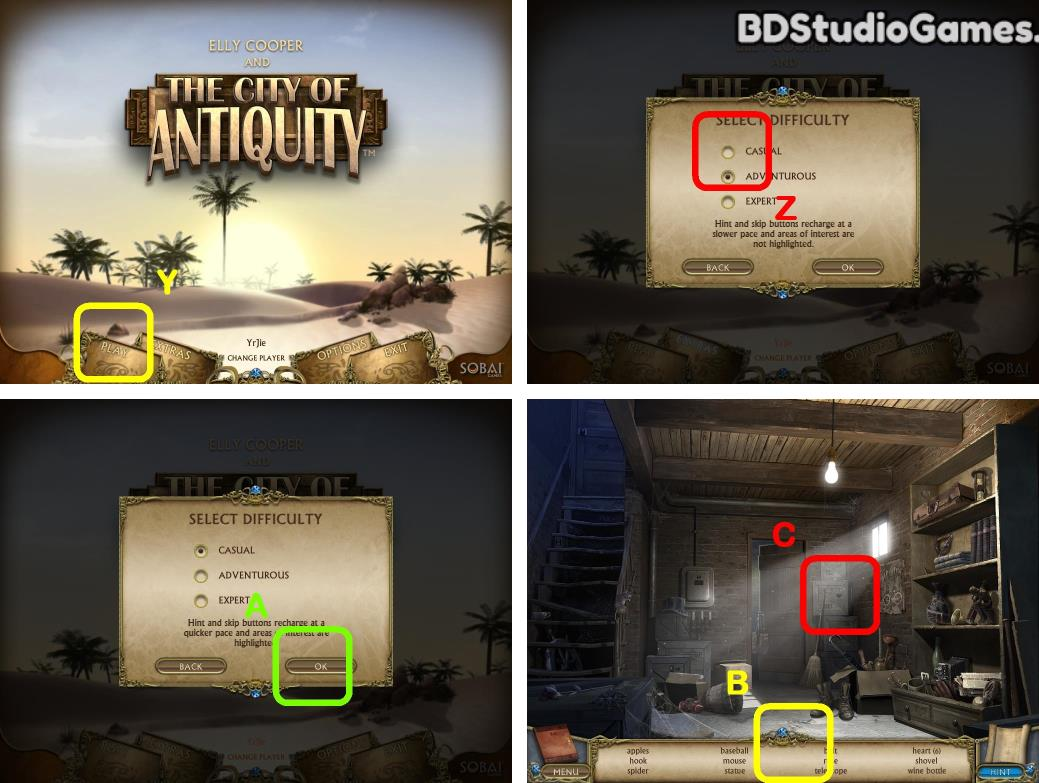Find all hidden objects. (B)
Take it. (C)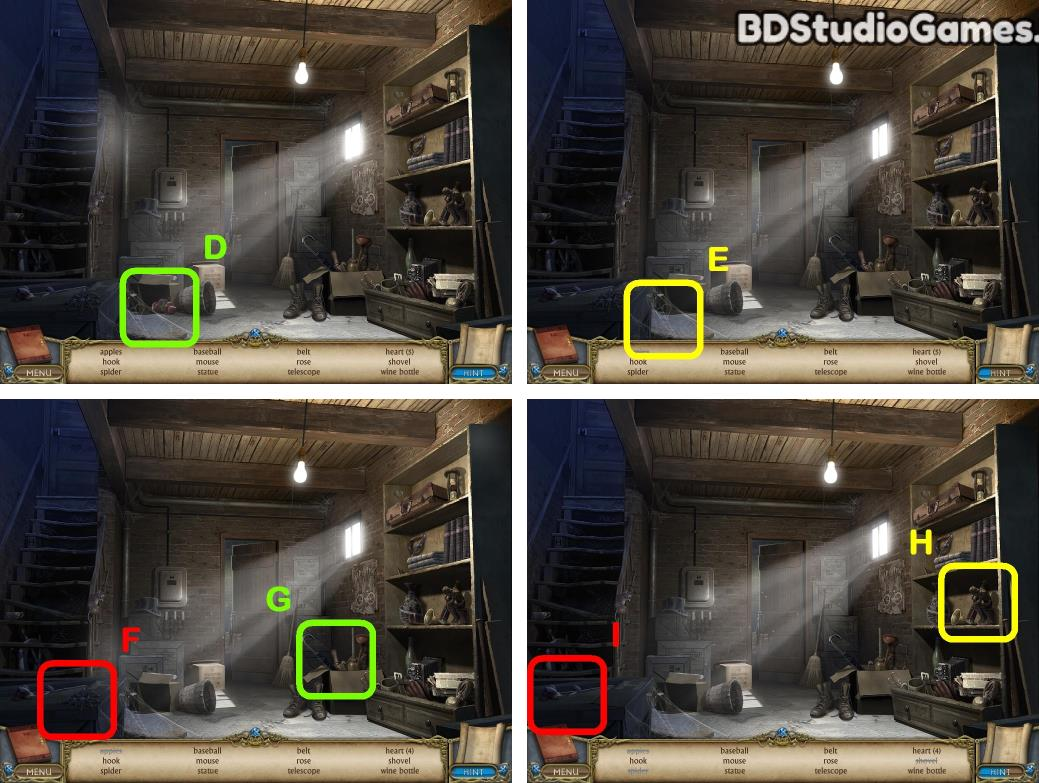Take it. (D)
Take it. (E)
Take it. (F)
Take it. (G)
Take it. (H)
Take it. (I)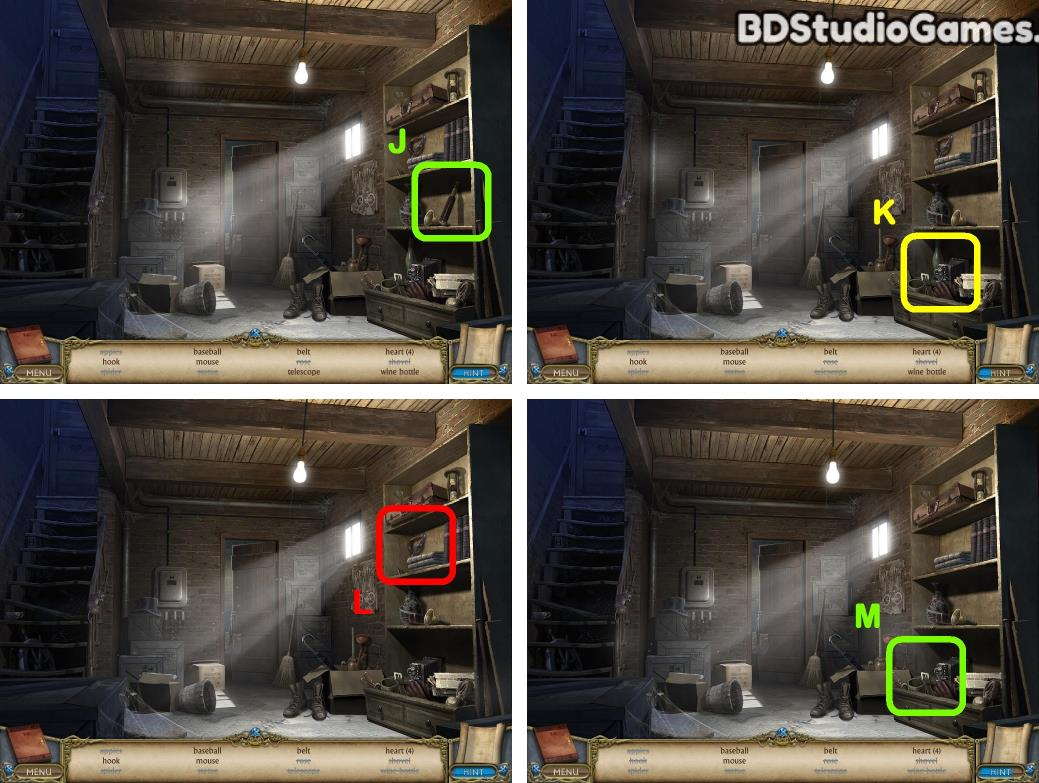Take it. (J)
Take it. (K)
Take it. (L)
Take it. (M)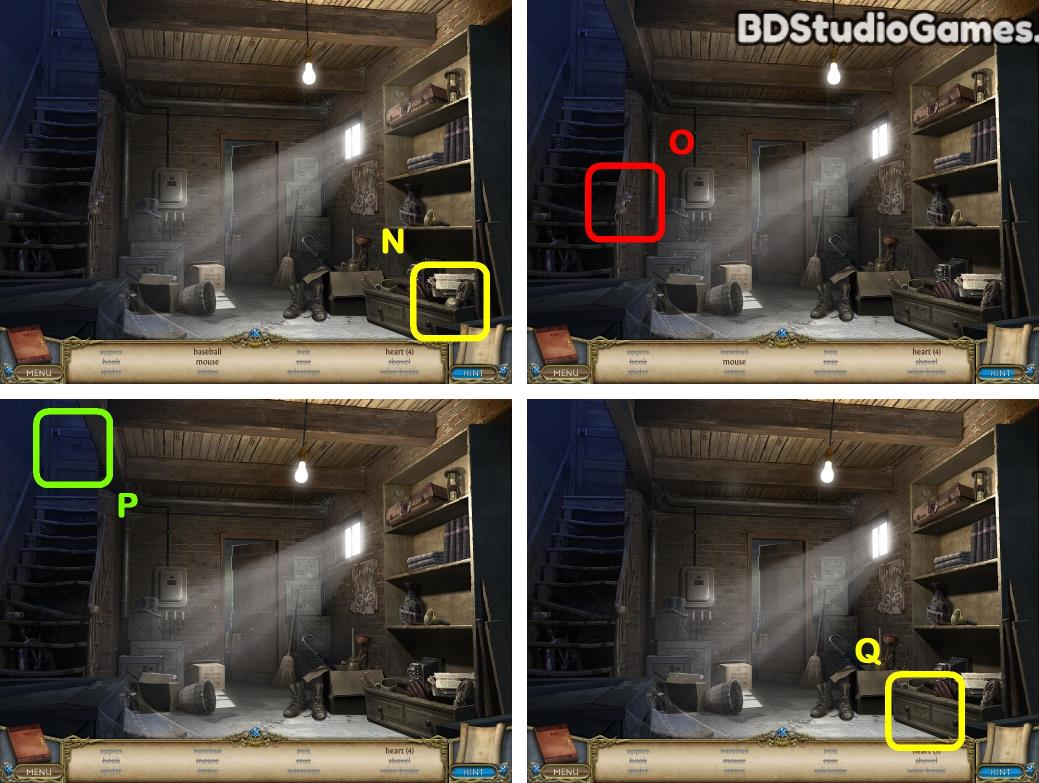Take it. (N)
Take it. (O)
Take it. (P)
Take it. (Q)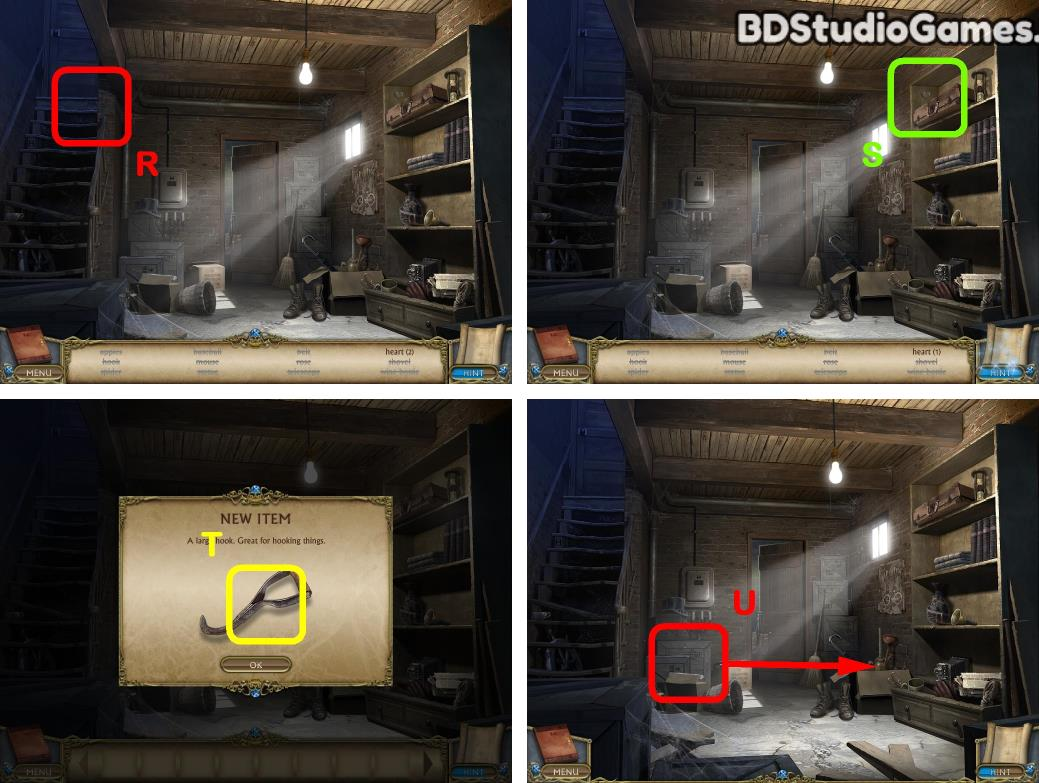Take it. (R)
Take it. (S)
Take it. (T)
Use object on here. (U)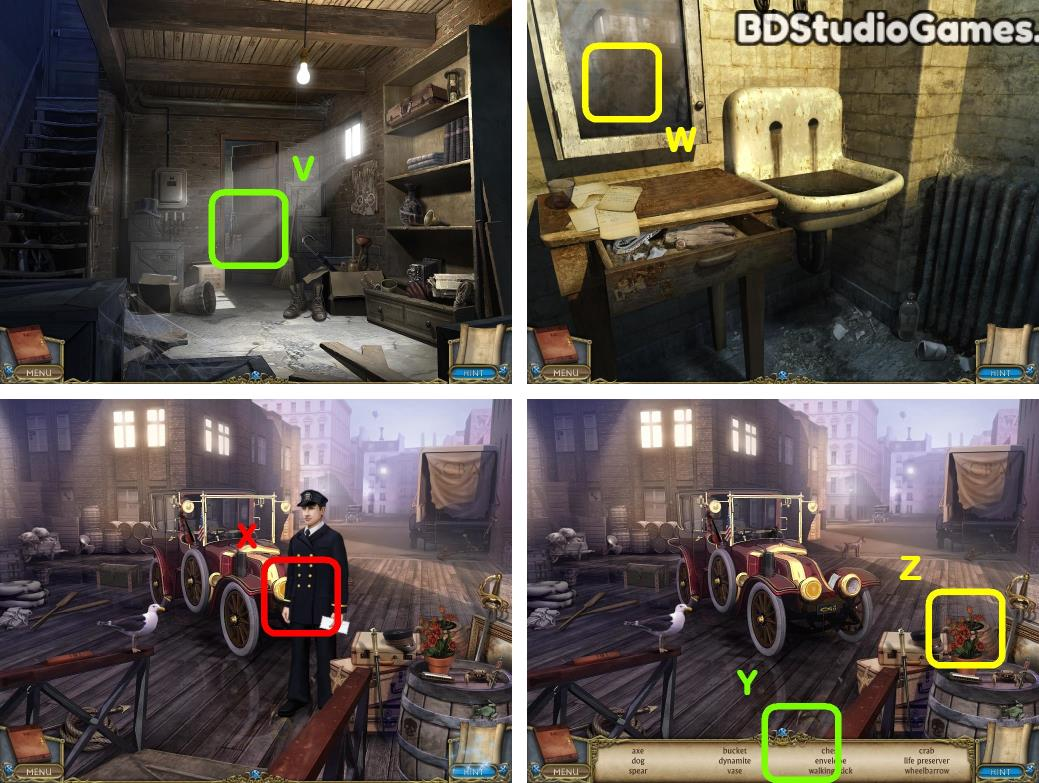Go Here. (V)
Examine here. (W)
Find all hidden objects. (Y)
Take it. (Z)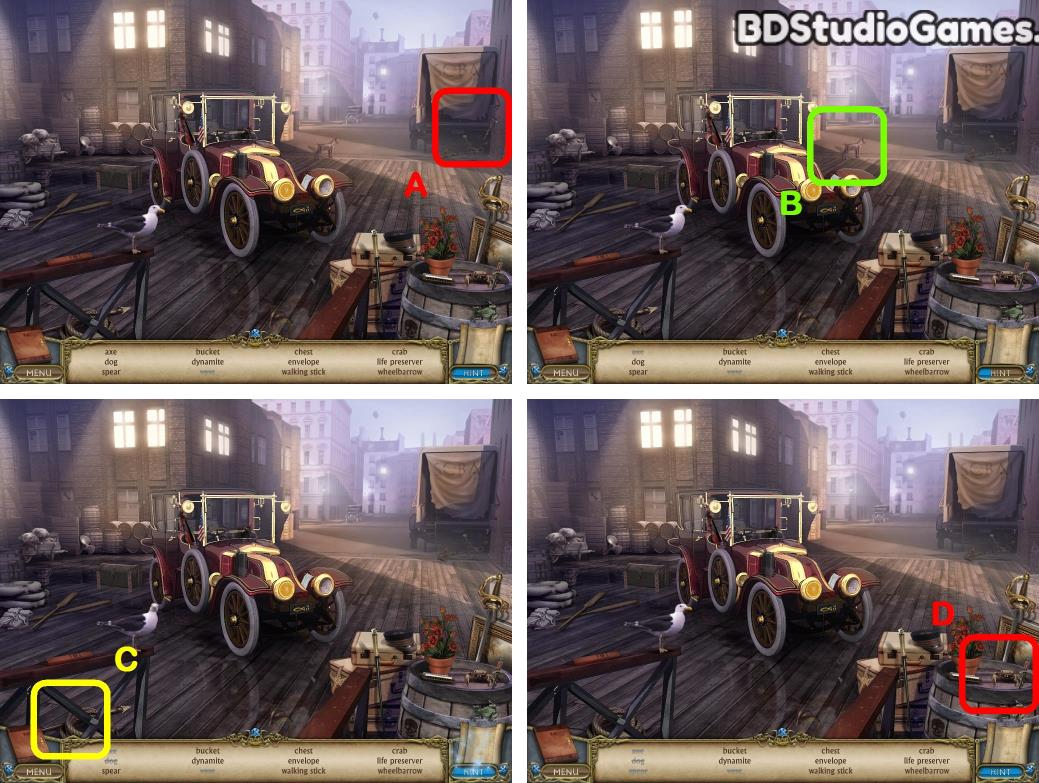Take it. (A)
Take it. (B)
Take it. (C)
Take it. (D)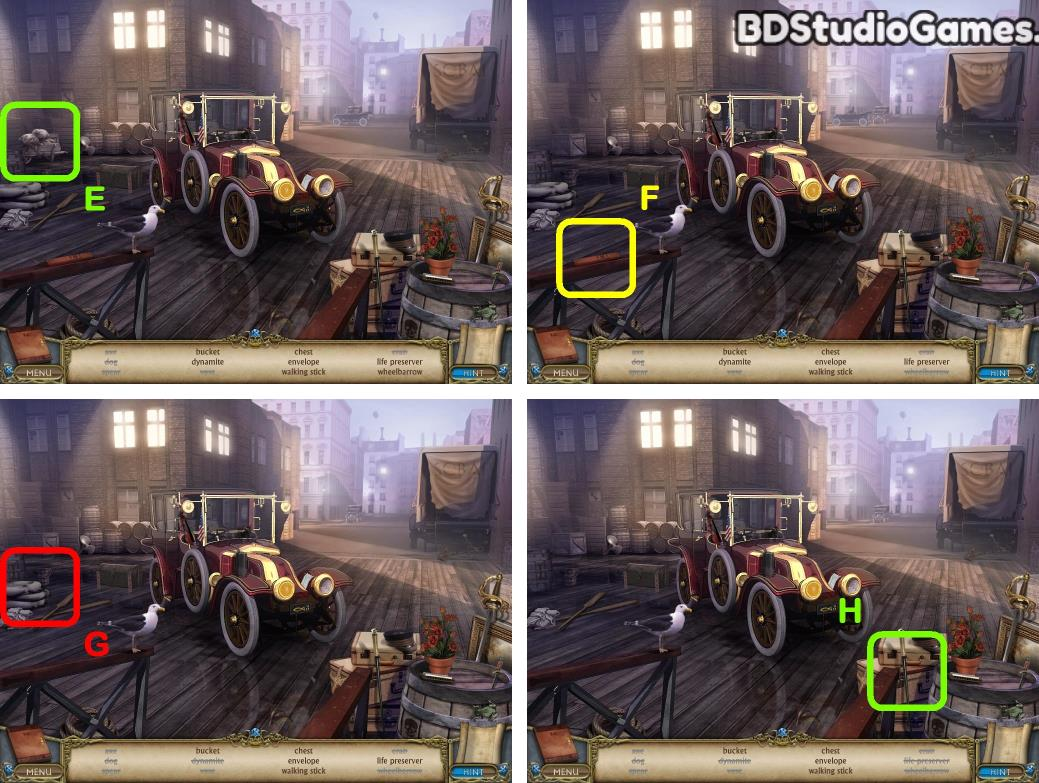Take it. (E)
Take it. (F)
Take it. (G)
Take it. (H)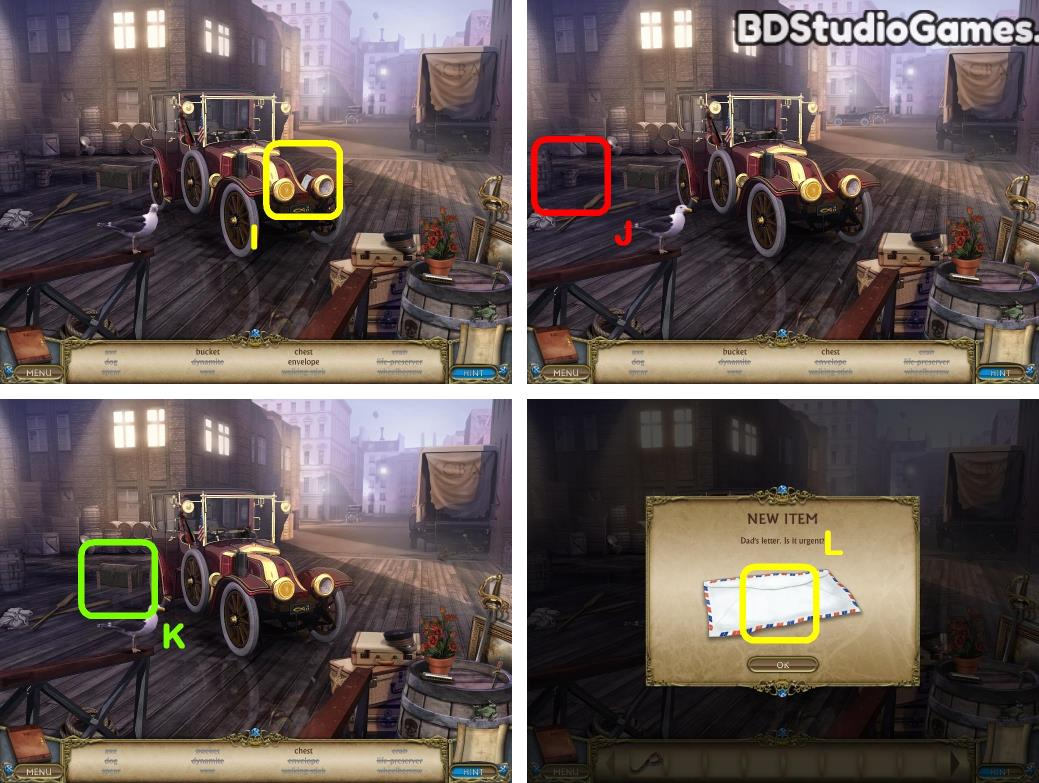Take it. (I)
Take it. (J)
Take it. (K)
Take it. (L)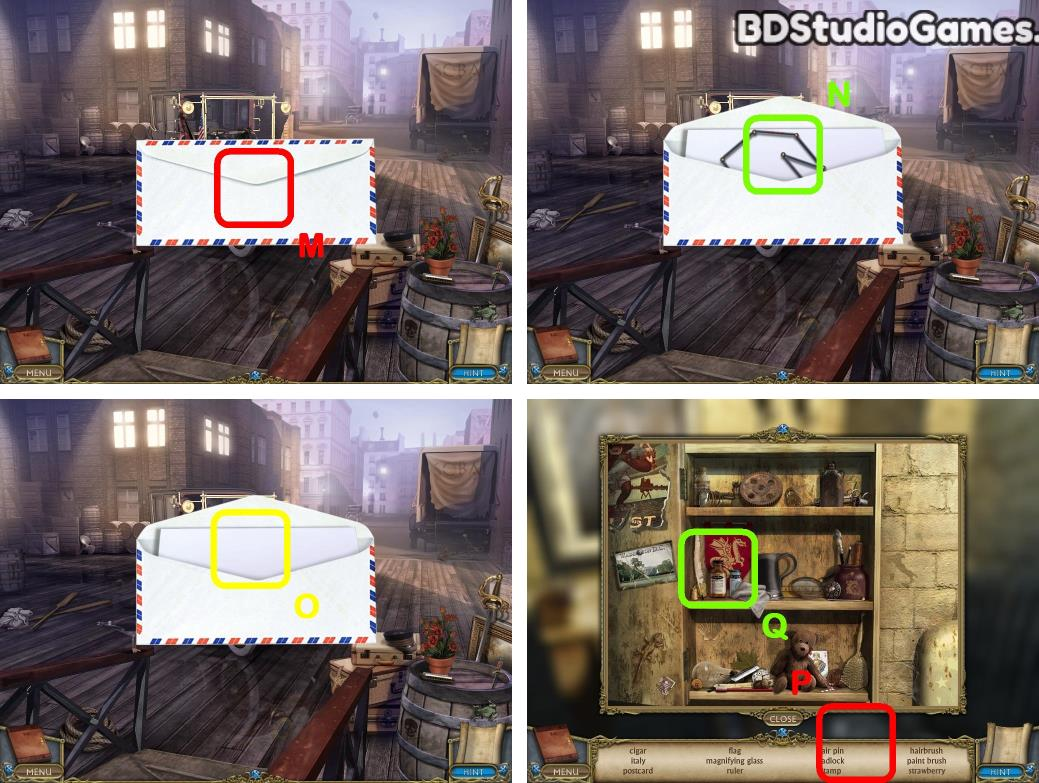Take it. (N)
Find all hidden objects. (P)
Take it. (Q)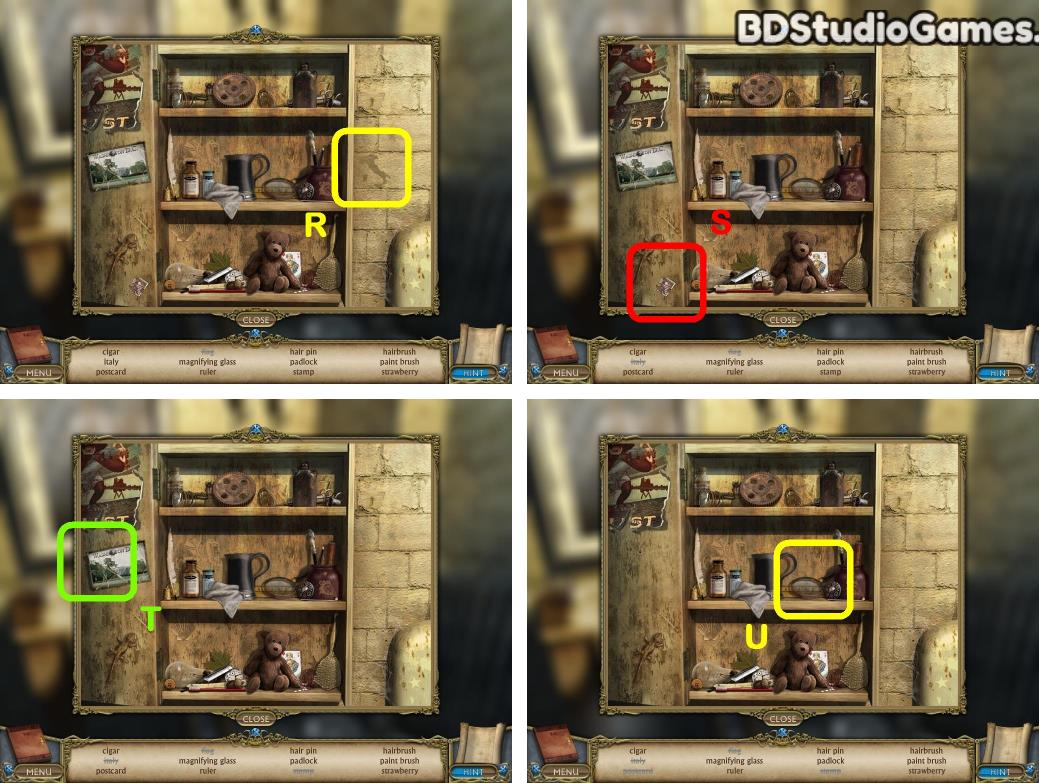Take it. (R)
Take it. (S)
Take it. (U)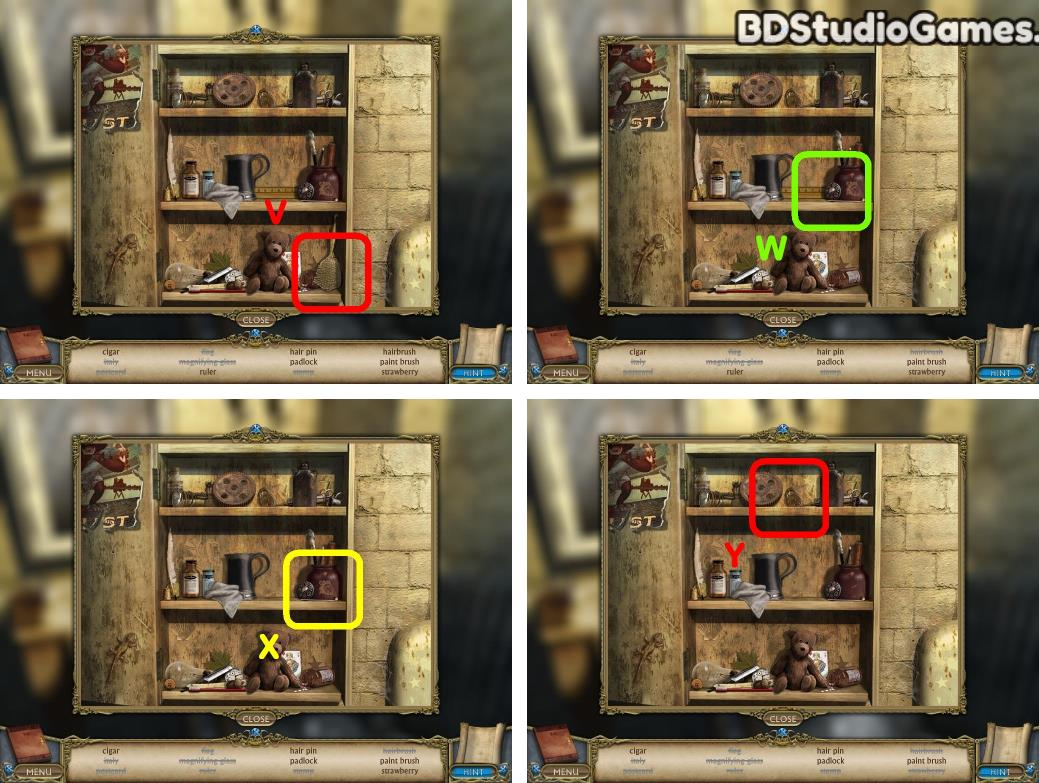Take it. (W)
Take it. (X)
Take it. (Y)Take it. (Z)
Take it. (C)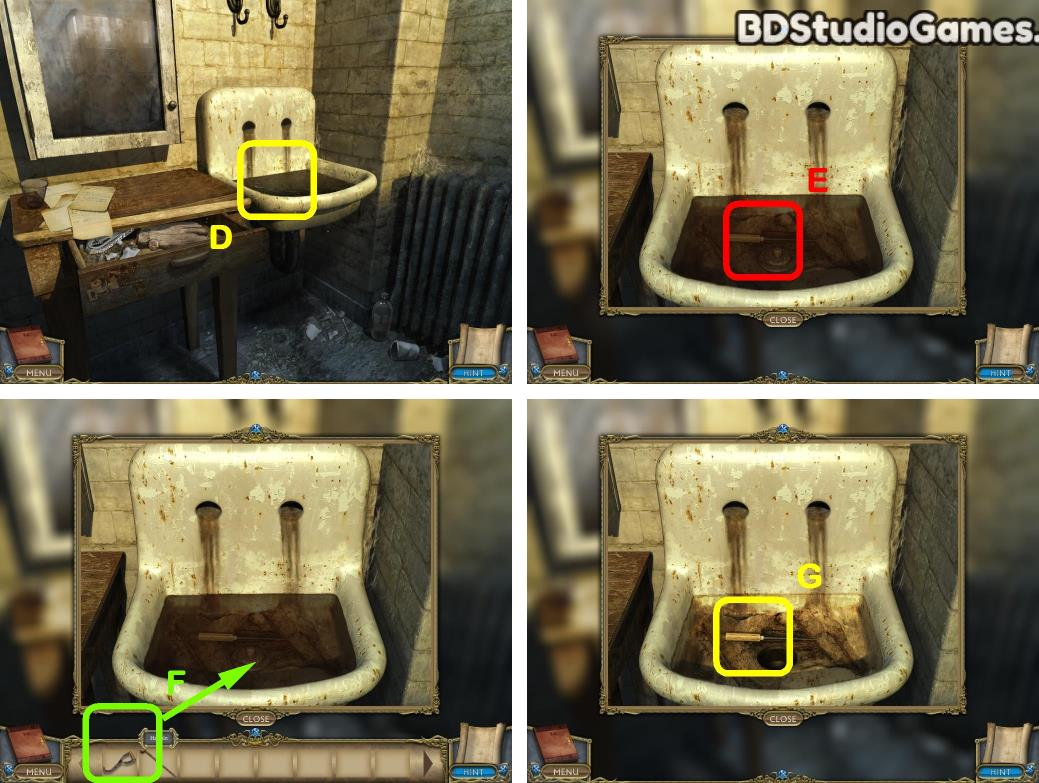Examine here. (D)
Use object on here. (F)
Take it. (G)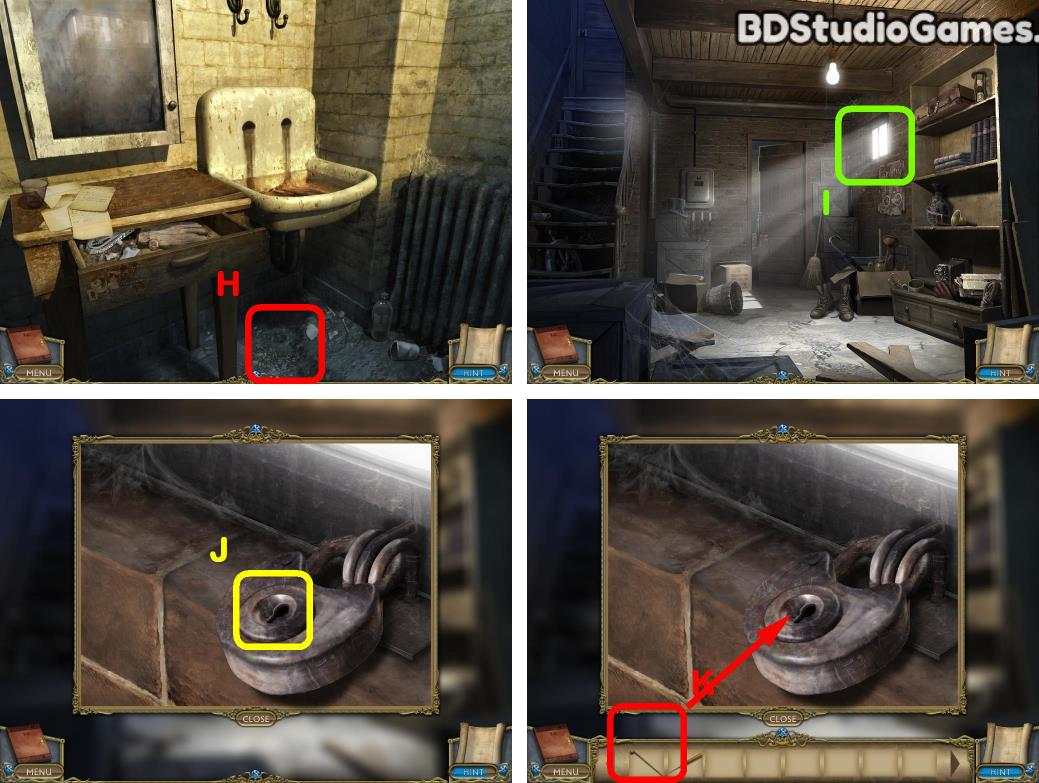Go Here. (H)
Examine here. (I)
Use object on here. (K)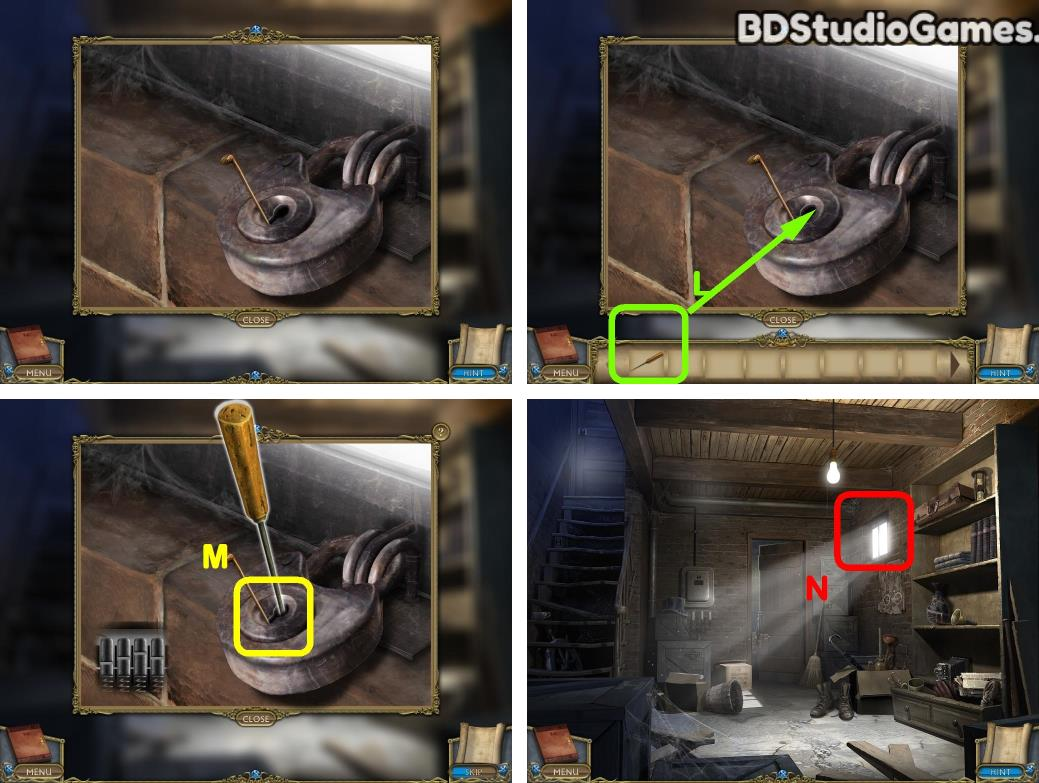Use object on here. (L)
Solve the puzzle. (M)
Go Here. (N)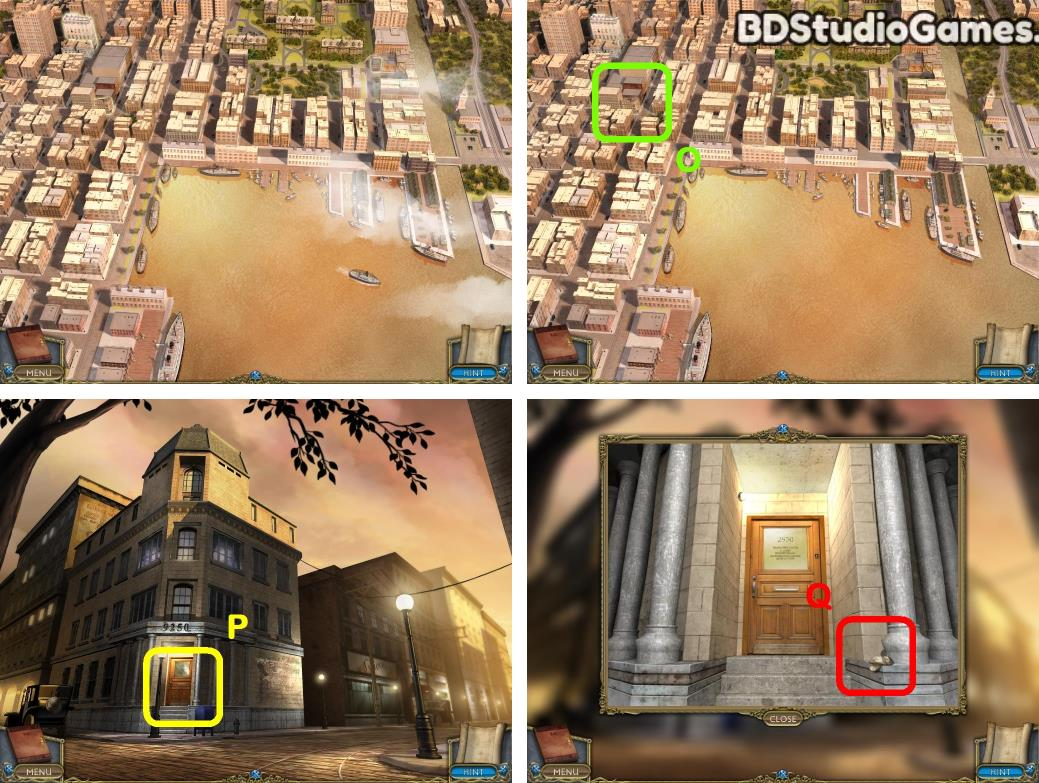Examine here. (O)
Examine here. (P)
Take it. (Q)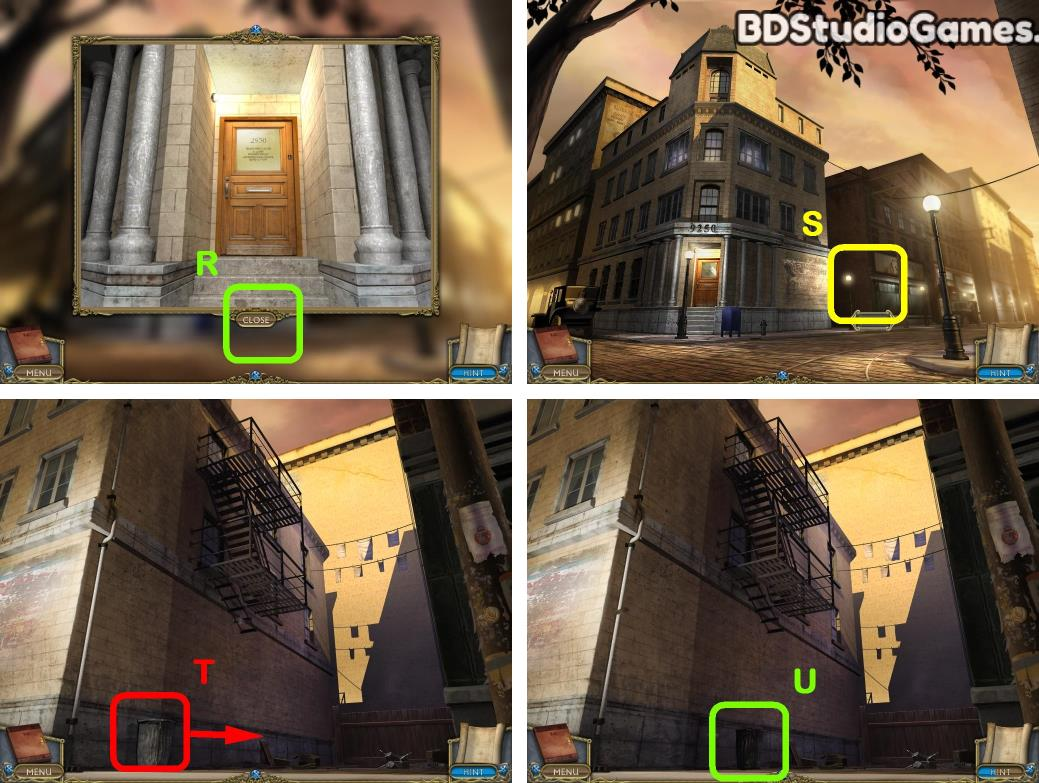Go Here. (S)
Use object on here. (T)
Examine here. (U)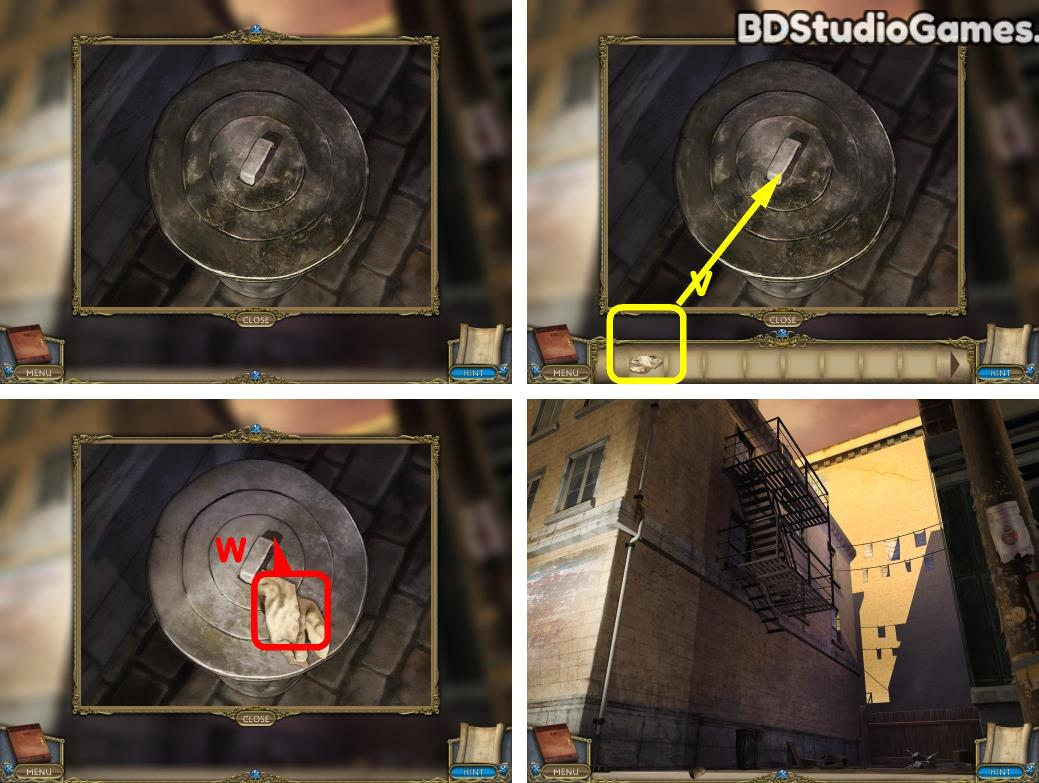Use object on here. (V)
Use object on here. (W)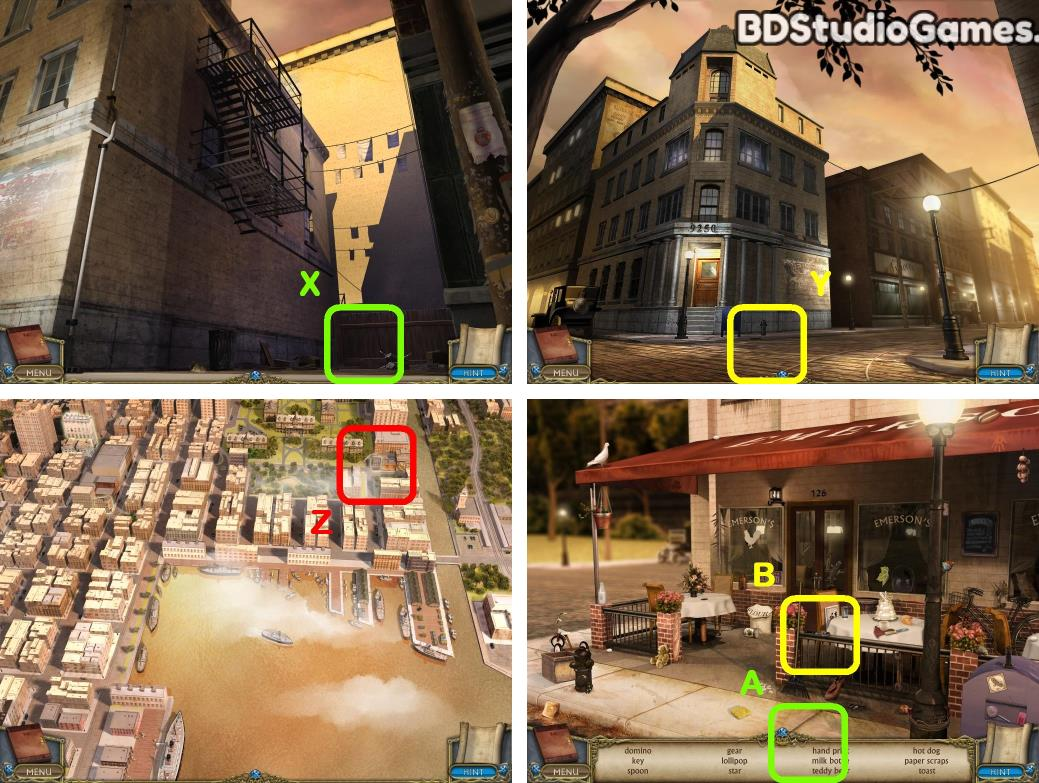Go Here. (X)
Go Here. (Y)
Examine here. (Z)
Find all hidden objects. (A)
Take it. (B)